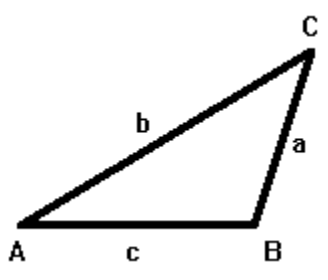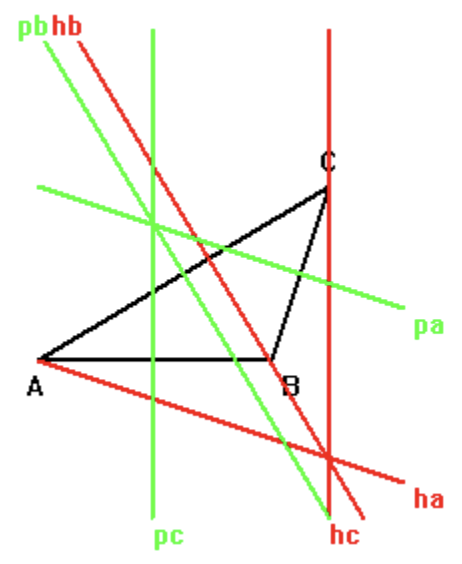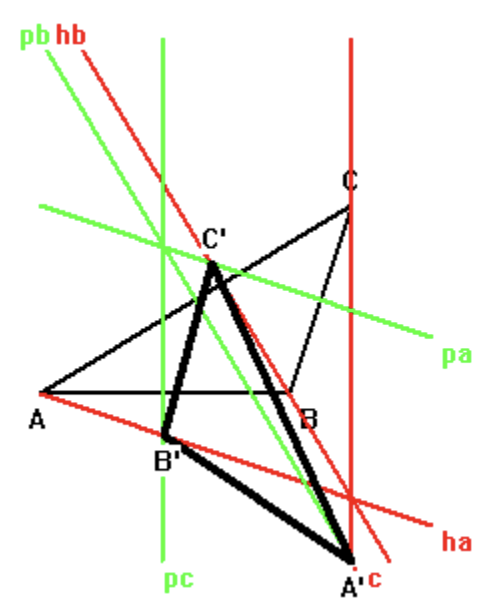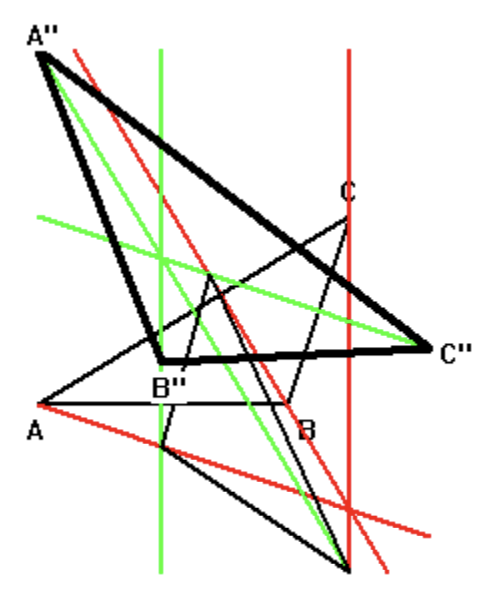시간 제한 메모리 제한 제출 정답 맞은 사람 정답 비율
1 초 512 MB 5 3 3 60.000%

## 문제

Starting with a △ABC with vertices A, B, C and sides a, b, c opposite A, B, C, respectively:Construct the altitudes ha, hb and hc to sides a, b, c respectively (red) and the perpendicular bisectors pa, pb and pc to sides a, b, c (green):Let A’ = hc intersect pb, B’ = ha intersect pc and C’ = hb intersect pa yeilding a new △A’B’C’ similar to ABC as shown on the below.Finally, let A’’ = reflection of A’ in side b, B’’ = reflection of B’ in side c and C’’ = reflection of C’ in side a to obtain yet another similar triangle:Write a program which takes as input the coordinates of the vertices A, B and C and outputs the areas of the three triangles.

## 입력

Input consists of a single line which contains three, space separated floating point values Bx, Cx, Cy in that order (-1.0 ≤ Bx, Cx, Cy ≤ 10.0). △ABC will have area at least 1.0. The coordinates system is chosen so that A = (0, 0) is the origin and B = (Bx, 0) lies on the X-axis. C = (Cx, Cy) is arbitrary.

## 출력

The output consists of a single line that contains three space separated floating point values to 4 decimal places. Area(△ABC), Area(△A’B’C’) and Area(△A”B”C”) in that order.

## 예제 입력 1

4.0 5.00 3.000


## 예제 출력 1

6.0000 4.8750 10.8750